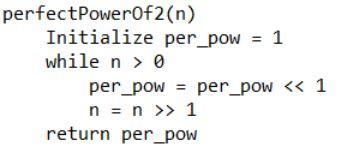GeeksforGeeks App
Open AppBrowser
Continue

# Smallest perfect power of 2 greater than n (without using arithmetic operators)

Given a non-negative integer n. The problem is to find the smallest perfect power of 2 which is greater than n without using the arithmetic operators.
Examples :

```Input : n = 10
Output : 16

Input : n = 128
Output : 256```

Algorithm :## C++

 `// C++ implementation of smallest perfect power``// of 2 greater than n``#include ` `using` `namespace` `std;` `// Function to find smallest perfect power``// of 2 greater than n``unsigned ``int` `perfectPowerOf2(unsigned ``int` `n)``{``    ``// To store perfect power of 2``    ``unsigned ``int` `per_pow = 1;` `    ``while` `(n > 0)``    ``{``        ``// bitwise left shift by 1``        ``per_pow = per_pow << 1;` `        ``// bitwise right shift by 1``        ``n = n >> 1;``    ``}` `    ``// Required perfect power of 2``    ``return` `per_pow;``}` `// Driver program to test above``int` `main()``{``    ``unsigned ``int` `n = 128;``    ``cout << ``"Perfect power of 2 greater than "``        ``<< n << ``": "` `<< perfectPowerOf2(n);``    ``return` `0;``}`

## Java

 `// JAVA Code for Smallest perfect``// power of 2 greater than n``import` `java.util.*;` `class` `GFG {``    ` `    ``// Function to find smallest perfect``    ``// power of 2 greater than n``    ``static` `int` `perfectPowerOf2( ``int` `n)``    ``{``        ``// To store perfect power of 2``         ``int` `per_pow = ``1``;``     ` `        ``while` `(n > ``0``)``        ``{``            ``// bitwise left shift by 1``            ``per_pow = per_pow << ``1``;``            ` `            ``n = n >> ``1``;``        ``}``     ` `        ``// Required perfect power of 2``        ``return` `per_pow;``    ``}``    ` `    ``// Driver program``    ``public` `static` `void` `main(String[] args)``    ``{``         ``int` `n = ``12``;``         ``System.out.println(``"Perfect power of 2 greater than "``                            ``+ n + ``": "` `+ perfectPowerOf2(n));``        ``}``    ``}``        ` `    ``//This code is contributed by Arnav Kr. Mandal.`

## Python3

 `# Python3 implementation of smallest``# perfect power of 2 greater than n` `# Function to find smallest perfect``# power of 2 greater than n``def` `perfectPowerOf2( n ):``    ` `    ``# To store perfect power of 2``    ``per_pow ``=` `1``    ` `    ``while` `n > ``0``:``    ` `        ``# bitwise left shift by 1``        ``per_pow ``=` `per_pow << ``1``        ` `        ``# bitwise right shift by 1``        ``n ``=` `n >> ``1``        ` `    ``# Required perfect power of 2``    ``return` `per_pow` `# Driver program to test above``n ``=` `128``print``(``"Perfect power of 2 greater than"``,``            ``n, ``":"``,perfectPowerOf2(n))` `# This code is contributed by "Sharad_Bhardwaj".`

## C#

 `// C# Code for Smallest perfect``// power of 2 greater than n``using` `System;` `class` `GFG {` `    ``// Function to find smallest perfect``    ``// power of 2 greater than n``    ``static` `int` `perfectPowerOf2(``int` `n)``    ``{``        ``// To store perfect power of 2``        ``int` `per_pow = 1;` `        ``while` `(n > 0)``        ``{``            ``// bitwise left shift by 1``            ``per_pow = per_pow << 1;` `            ``n = n >> 1;``        ``}` `        ``// Required perfect power of 2``        ``return` `per_pow;``    ``}` `    ``// Driver program``    ``public` `static` `void` `Main()``    ``{``        ``int` `n = 128;``        ``Console.WriteLine(``"Perfect power of 2 greater than "` `+``                           ``n + ``": "` `+ perfectPowerOf2(n));``    ``}``}` `// This code is contributed by Sam007`

## PHP

 ` 0)``    ``{``        ``// bitwise left shift by 1``        ``\$per_pow` `= ``\$per_pow` `<< 1;` `        ``// bitwise right shift by 1``        ``\$n` `= ``\$n` `>> 1;``    ``}` `    ``// Required perfect power of 2``    ``return` `\$per_pow``;``}` `    ``// Driver code``    ``\$n` `= 128;``    ``echo` `"Perfect power of 2 greater than "``.``          ``\$n` `. ``": "``.perfectPowerOf2(``\$n``);` `// This code is contributed by mits``?>`

## Javascript

 ``

Output:

`Perfect power of 2 greater than 128: 256`

Time Complexity: O(logn)
Auxiliary Space: O(1)

My Personal Notes arrow_drop_up